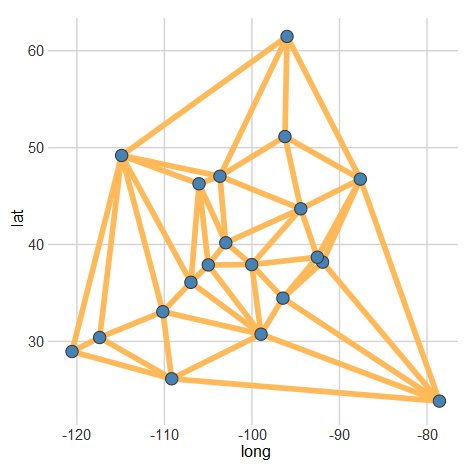# Creating Voronoi Diagrams with ggplot

A Voronoi diagram (or tessellation) is neat way of visualizing spatial data. It essentially allows us to see the areas that are closest to a set of locations. For example, this map shows all of the Criagslist localities and the regions closest to each Craigslist locality. More formally, “A set of points (called seeds, sites, or generators) is specified beforehand and for each seed there will be a corresponding region consisting of all points closer to that seed than to any other.” (Wikipedia)

A handy library for plotting these in R is deldir. After installing that library (and ggplot2 if you don’t already have it), plotting a Voronoi diagram is simple.

```#Let's generate some fake data
set.seed(105)
long<-rnorm(20,-98,15)
lat<-rnorm(20,39,10)
df <- data.frame(lat,long)

library(deldir)
library(ggplot2)

#This creates the voronoi line segments
voronoi <- deldir(df\$long, df\$lat)

#Now we can make a plot
ggplot(data=df, aes(x=long,y=lat)) +
#Plot the voronoi lines
geom_segment(
aes(x = x1, y = y1, xend = x2, yend = y2),
size = 2,
data = voronoi\$dirsgs,
linetype = 1,
color= "#FFB958") +
#Plot the points
geom_point(
fill=rgb(70,130,180,255,maxColorValue=255),
pch=21,
size = 4,
color="#333333") +
#(Optional) Specify a theme to use
ltd_theme
```

You should end up with something like this:Additionally, if you would prefer the Delaunay triangulation (which connects all of the sites), you just need to change `voronoi\$dirsgs` to `voronoi\$delsgs` in the line segment part of the plot. Here’s what that should look like:I have not found a fully automated solution to combine these plots with a map. However, using the ggmap library can get you pretty close.

Tagged on: , , , , ,

### 8 thoughts on “Creating Voronoi Diagrams with ggplot”

1.PAC

I think that in the first graph, you’ve plotted voronoi\$dirsgs instead of voronoi\$delsgs.

Would you know how to create polygons instead of segments ?

1.Phillip

Thanks, updated.

ggplot2 has a plotting option for polygons. You should be able to extract the points from delsgs or dirsgs and then reshape them as described in the ggplot2 documentation page. Here’s a quick overview of the deldir package that may help sorting out what data is available to you.

2.arvi1000

Helpful post and comments, thanks! The deldir lib turned out to be just what I was looking for this afternoon

3.Al

This example is really clear!
But how to add ID labels
to each blue “node”
(ie: “A”, “B”, “C”…).

With a label (ID)
for each blue node ,
this code would really be more useful
in a real-world application…

What would be the R code?

Thanks!

1.Ray Al

Thank you, Phillip!
Done.

Reason for my question:
Imagine you have 26 cell antennas,
each labeled with a single, unique letter:
A to Z.

Ideally, at any time
given your mobile location (latitude, longitude)
you’d like to use the antenna closest to your location.
Take a peek!
your example code with deldir and ggplot2:

```#Let's generate some fake data
set.seed(105)
long<-rnorm(26,-98,15)
lat<-rnorm(26,39,10)
df <- data.frame(lat,long,name=c(LETTERS))
library(deldir)
library(ggplot2)
#This creates the voronoi line segments
voronoi <- deldir(df\$long, df\$lat)
#Now we can make a plot
ggplot(data=df, aes(x=long,y=lat)) +

#Plot the voronoi lines
geom_segment(
aes(x = x1, y = y1, xend = x2, yend = y2),
size = 1,
data = voronoi\$dirsgs,
# or for Delaunay lines btw pts., use: data=voronoi\$delsgs
linetype = 1,
color= "#FFB958") +
geom_text(aes(label=df\$name),hjust=-0.5, vjust=0) +

#Plot the points
geom_point(
fill=rgb(70,130,180,255,maxColorValue=255),
pch=21,
size = 2,
color="#333333")
```

So, from this generated Voronoi diagram,
you can visually "see" that a location:
long= -110 and lat = 40
places you in the region with the R antenna.
That's the closest antenna to where you are now.

But, the question is…
without looking at this Voronoi diagram,
how to input this (lat, long) location to deldir()
to simply get the value:
"R"
as an output antenna result?
(computationally, not visually…).

Is there a deldir() option/parameter for this?